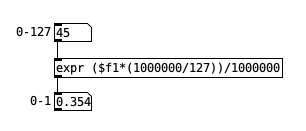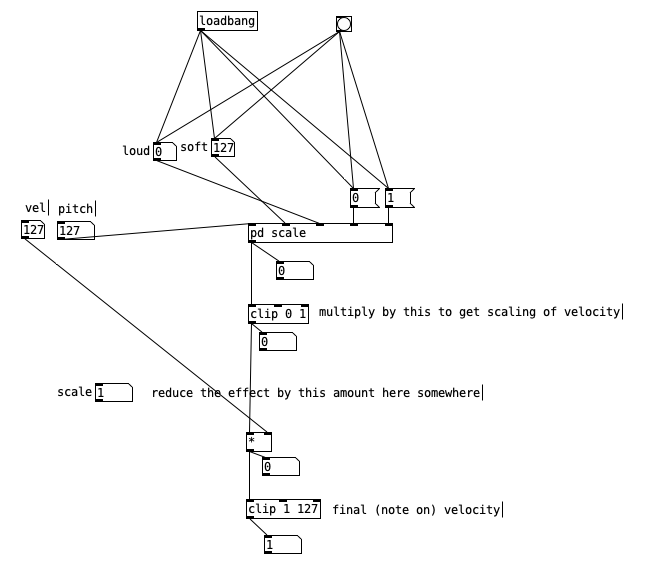• ### Scale something to something pretty and efficient

Hi,

I am looking for simple and efficient way of rescaling say 0-127 to 0-1, or 0-100. Also, how one could minimize mismatch. I find no object to do this.

I would do it like this:miditopercent.pd

But this never reaches 1.

How do you usually do it?

I though I saw something on this in the documentation(s) but I can not find it.

• Posts 8 | Views 863
• @cfry scale.zip
Abstraction using [expr] from the jmmmp library.
David.

• I don't get it,, why not just multiply by the reciprocal of 127 (~0.00787402) using `[* ]`? (multiplication is more efficient than division I think)
To get 0-100 just multiply by 100/127=0.78740157480315

• Because `\$f1` is already floating point, you gain nothing by multiplying by 1000000. That might be useful in the integer domain, but floating point numbers "shift" their full precision based on the magnitude, so it's a wasted operation.

Then, dividing by 1000000 is the same as multiplying by 0.000001. These are convenient numbers in decimal, but they are repeating fractions in binary floating point (like 1/7 = 0.142847142847...) so... you thought multiplying and dividing would give you extra precision but in fact, you've just introduced extra rounding error. This probably explains why it never reaches 1. (This is a very common misconception with floating point numbers. We tend to assume that a finite decimal should also be a finite float, but computer floats are binary. Any rational number where the denominator's prime factors are 2 and 5 will be finite in decimal, but a factor of 5 in the denominator will create an infinitely repeating binary representation, so 0.1 decimal is 1.1001100110011... x 2^(-4) in binary -- it's no more precise than 0.3333 in decimal or binary.)

hjh

• thanks all, that set me straight.

• I adapted that abstraction to make an attenuator of loudness depending on midi pitch, using the scaling parameters to set a start and stop point of the scaling. But the I just got stuck on the easiest part, the control the amount of scaling. Brain freeze.midiscaler.pd

When the midi pitch reaches the "soft" value the reduction of velocity should be in the range of no reduction (original velocity) to full reduction (velocity 1) depending on the setting (amount/scale/attenuation whatever the best name would be).

:/

• @cfry maybe.zip
It depends.
The notes will get quieter as they fall in pitch, but less so if the "amount" fader is moved toward "less".
I have added the same feature to the velocity just in case.
David,

• @whale-av
thanks, that [expr_scale f f f f] object is golden, with a few edits it now works perfect.

Posts 8 | Views 863
Internal error.

Oops! Looks like something went wrong!